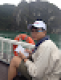# Can you solve a math problem: x+y=42, x-y=30?In page 43, math problem 1, on Math 4 Workbook, Part One, Vietnamese Education Publishing House:
The sum of mother and son's ages is 42. The mother is 30 years older than her son. How old is the mother and her son?

edit retag close merge delete

Try something like:

sage: var('x,y');
sage: solve([x + y == 42, x - y == 30], [x, y])
[[x == 36, y == 6]]


(Or just ignore sage code, and use plain thoughts or simple drawings.)

Sort by » oldest newest most voted

Sage is able to solve such systems of equations, you can have a look at the tutorial : https://doc.sagemath.org/html/en/tuto...

more